# Logic Gates

## Homework Statement

Make the design of a circuit returning the absolute value of a number of 3 bits . The input and output
must be signed , use the complement 2. Show your approach and draw your track
using logic gates.

I seriously do not understand this at all. What am I supposed to do?

N/A

N/A

phinds
Gold Member
2021 Award

## Homework Statement

Make the design of a circuit returning the absolute value of a number of 3 bits . The input and output
must be signed , use the complement 2. Show your approach and draw your track
using logic gates.

I seriously do not understand this at all. What am I supposed to do?

N/A

## The Attempt at a Solution

N/A

What is your background? How much have you studied logic and/or digital electronics? What kind of course is this for? How is it that you are being asked to do a problem and you don't even understand the problem, much less how to solve it?

What is your background? How much have you studied logic and/or digital electronics? What kind of course is this for? How is it that you are being asked to do a problem and you don't even understand the problem, much less how to solve it?
This is electronic engineer first year Bach. Its a course about Digital electronics, we just started 1 month ago. I understand logic, gates, etc. I just do not understand what I actually have to do with the question, for me its not clear enough.

Some step on how I have to proceed would actually help me.

How I do the table of truth of it?

circuit returning the absolute value of a number of 3 bits // returning the absolute value??? what does this mean? How can it be negative and return the absolute value at the same time if we want the output to be signed(negative)?
The input and output must be signed // signed? what does this actually mean, It must be negative?
use the complement 2 // I know what is complement 2.

This is all confusing me.

phinds
Gold Member
2021 Award
This is electronic engineer first year Bach. Its a course about Digital electronics, we just started 1 month ago. I understand logic, gates, etc. I just do not understand what I actually have to do with the question, for me its not clear enough.

Some step on how I have to proceed would actually help me.

How I do the table of truth of it?
Well, can you write out all the possible numbers using 3 digits of two's complement notation? That's the first step. Then show what each of them have to be to represent the absolute value of the same number. Can you do that?

Well, can you write out all the possible numbers using 3 digits of two's complement notation? That's the first step. Then show what each of them have to be to represent the absolute value of the same number. Can you do that?
Here:
A B C
0 0 0 = 0
0 0 1 = 1
0 1 0 = 2
0 1 1 = 3
1 0 0 = -4
1 0 1 = -3
1 1 0 = -2
1 1 1 = -1

Well, can you write out all the possible numbers using 3 digits of two's complement notation? That's the first step. Then show what each of them have to be to represent the absolute value of the same number. Can you do that?
What I have to do after this?
Thanks for helping me I really appreciate it.

phinds
Gold Member
2021 Award
Well, you've only answered half my question. You need to put what you have in a table and add what they need to become to be the absolute value. Then you have a pair of goals ... build the adder to get the first set then use logic gates to convert it to the second set. Better still would be to figure how to just combine the two.

Well, you've only answered half my question. You need to put what you have in a table and add what they need to become to be the absolute value. Then you have a pair of goals ... build the adder to get the first set then use logic gates to convert it to the second set. Better still would be to figure how to just combine the two.
Can you give me an example of this please "You need to put what you have in a table and add what they need to become to be the absolute value."
I don't really understand.

phinds
Gold Member
2021 Award
Do you know what "absolute value" IS? You have a value of +3 and you have a value for -3. How would the two relate when taking the absolute value of each?

Do you know what "absolute value" IS? You have a value of +3 and you have a value for -3. How would the two relate when taking the absolute value of each?

A B C Absolute value
0 0 0 = 0 0 0 0
0 0 1 = 1 0 0 1
0 1 0 = 2 0 1 0
0 1 1 = 3 0 1 1
1 0 0 = -4 1 0 0
1 0 1 = -3 0 1 1
1 1 0 = -2 0 1 0
1 1 1 = -1 0 0 1

would this be correct?

phinds
Gold Member
2021 Award
Good. Now can you map the input to the output. That is, skip the adder step and just write down the inputs and the outputs to your circuit.

Good. Now can you map the input to the output. That is, skip the adder step and just write down the inputs and the outputs to your circuit.
Just so I understand better.

I have 3 intput and 3 output right?

For example my input is A B C, and I have 1 1 1 as my input, my output should be 0 0 1?
So to do that I have to make a logic circuit with AND / OR /NOT ?

phinds
Gold Member
2021 Award
Just so I understand better.

I have 3 intput and 3 output right?

For example my input is A B C, and I have 1 1 1 as my input, my output should be 0 0 1?
So to do that I have to make a logic circuit with AND / OR /NOT ?
Yes, although what type(s) of gate(s) you use is sometimes a part of the problem statement. Since that does not seem to be the case here, then yes, I would stick to AND / OR / NOT types

So map the 3-bit input to the 3-bit output. Do you know how to use Karnaugh maps? That would be my personal preference for the next step but it's not the only way to go.

Yes, although what type(s) of gate(s) you use is sometimes a part of the problem statement. Since that does not seem to be the case here, then yes, I would stick to AND / OR / NOT types

So map the 3-bit input to the 3-bit output. Do you know how to use Karnaugh maps? That would be my personal preference for the next step but it's not the only way to go.
I understand Karnaugh but in my case, I don't understand how to do it since I have 3 outputs.
Usually I do it with 1-5 inputs and 1 output only.

phinds
Gold Member
2021 Award
I understand Karnaugh but in my case, I don't understand how to do it since I have 3 outputs.
Usually I do it with 1-5 inputs and 1 output only.
Well, maybe you could do a K-map for EACH of the 3 outputs ... might that work?

Well, maybe you could do a K-map for EACH of the 3 outputs ... might that work?
So for the first Karnaugh would this be right? its for the first ouput.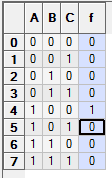phinds
Gold Member
2021 Award
So for the first Karnaugh would this be right? its for the first ouput.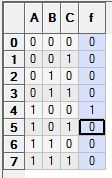Since you have not defined what "first output" means, I wouldn't know. Do you mean the most significant bit or the least significant bit? Do you see how that designation is more meaningful than "first output" ?

Since you have not defined what "first output" means, I wouldn't know. Do you mean the most significant bit or the least significant bit? Do you see how that designation is more meaningful than "first output" ?
---------------Outputs
A B C = ------1 2 3
0 0 0 = 0-----0 0 0
0 0 1 = 1-----0 0 1
0 1 0 = 2-----0 1 0
0 1 1 = 3-----0 1 1
1 0 0 = -4----1 0 0
1 0 1 = -3----0 1 1
1 1 0 = -2----0 1 0
1 1 1 = -1----0 0 1

Output1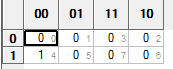Equation: A/B/C

Output2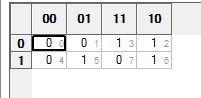Equation: /AB+A/BC+B/C

Output3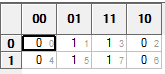Equation: C

To put all this together I do this ? C + (/AB+A/BC+B/C) + (A/B/C)

Last edited:
l

Last edited:
phinds
Gold Member
2021 Award
Your equations at a quick glance appear (1) wrong, and (2) ridiculously complex.

For example, #3 obviously should be 3=C

You K-maps look nothing like what I am accustomed to. Is that how they are done these days? Here's mine.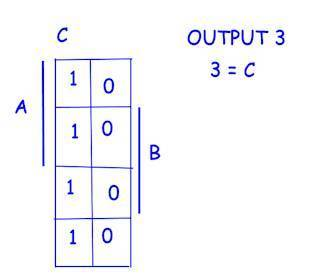NascentOxygen
Staff Emeritus
---------------Outputs
A B C = ------1 2 3
0 0 0 = 0-----0 0 0
0 0 1 = 1-----0 0 1
0 1 0 = 2-----0 1 0
0 1 1 = 3-----0 1 1
1 0 0 = -4----1 0 0
1 0 1 = -3----0 1 1
1 1 0 = -2----0 1 0
1 1 1 = -1----0 0 1

Alternatively, you could determine the logic by examining your table. Looking at output 2,
we see it's 1 when A=0,B=1,C=? and also when A=1,B=0,C=1 and when A=1,B=1,C=0
This gives rise to the equation: O2 = A' B + A B' C + A B C'
and maybe this can be simplified further.

By inspection, you can see that whenever C is 1 then O3 is 1, so we can write O3 = C, as phinds found.

Last edited:
One last question.
If I want to write -2 in binary in Sign&magnitude format, is 110 correct? I have to use the least bit possible.

phinds
Gold Member
2021 Award
The sign bit being on implies negative.

The sign bit being on implies negative.
So it would be right to say its 110? Because my teacher puts 1010 and says to use least bit possible.

NascentOxygen
Staff Emeritus
So it would be right to say its 110? Because my teacher puts 1010 and says to use least bit possible.
Well, 1xx will allow a negative range -1...-3 and 1xxx will allow -1...-7

phinds
Gold Member
2021 Award
So it would be right to say its 110? Because my teacher puts 1010 and says to use least bit possible.
Which part of "The sign bit being on implies negative." did you not understand?

Last edited:
NascentOxygen
Staff Emeritus